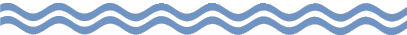The Rest of the Story:ax2 + bx + c

Now that you have conquered Lesson I of factoring trinomials (Trinomials I), it is time to tackle the next level:

ax2 + bx + c.

This means dealing with such expressions as

3x2 + 8x + 5

6x2 - 7x + 2

4x2 + 17x - 15

3x2 + 16x - 12

Right away, an observant student should recognize the similarity of these four cases with the four that were explained in Lesson I.### Background

Again, as I stated in the first lesson on trinomials, I feel much of a student's difficulty in factoring lies in the fact that there was not enough careful absorption of the patterns that were present when one was multiplying binomials. In the case before us, this means binomials of the form

### ax ± b

For the four examples cited above, the necessary binomials are the following:

(3x + 5)(x + 1)

(2x - 1)(3x - 2)

(4x - 3)(x + 5)

(3x + 2)(x - 6)Let's categorize the patterns of the binomials above in much the same way we did before, namely:

• a) (ax + b)(cx + d) <-- where both signs are "+"
• b) (ax - b)(cx - d) <-- where both signs are "-"
• c) (ax + b)(cx - d) <-- where the middle term comes out "+"
• d) (ax + b)(cx - d) <-- where the middle term comes out "-"

For our examples the numerical presentation for case "a" and case "b" looks like this:

```			3x  + 5		2x  - 1
x  + 1		3x  - 2
3x2 + 5x	        6x2 -  3x
3x + 5	    -  4x + 2
3x2 + 8x + 5    6x2 - 7x + 2
```

The remaining two cases "c" and "d":

```			4x - 3		3x  + 2
x + 5		 x  - 6
4x2 - 3x	        3x2 +  2x
20x  - 15	    - 18x - 12
4x2 + 17x - 15  3x2 - 16x - 12
```### Factoring the Trinomials

When it came to factoring trinomials like these during my own student days, I did two things that were not in the books. The books usually presented a guess-and-check approach, listing ALL the possible combinations of binomials and finding the products. And it always seemed that the correct one was given at the bottom of the list.

Case in point: Taken from an old Algebra 2 text is this...

Factor 10x2 + 11x + 3 completely.

```
(10x + 1)(x + 3) = 10x2 + 31x + 3
(10x + 3)(x + 1) = 10x2 + 13x + 3
(5x + 1)(2x + 3) = 10x2 + 17x + 3
(5x + 3)(2x + 1) = 10x2 + 11x + 3
```
See? Why is the correct one always the last one given???

Well, I never relished very much the time consumed in writing out all that stuff. I just reverted to my vertical format that I used in Lesson one, stripping away all the parentheses, the "x's", and even the signs, and did some "old fashioned scratchwork" on the side of my paper, doing as much mentally as I could. For the book's example, I might write it like this:

```	10   3		10   1		5   1		5   3
1   1		 1   3		2   3		2   1
3  10		 1  30		2  15		6   5
```

The vertical pairs above the lines are just the factor combinations for the "a-coefficient" and the "c" term, the constant.

The numbers below the line are simply the cross-products of the values above. And since the sign of the third term is positive in the trinomial, I know I merely need the sum of those two values. Hence, the case of 6 and 5 does nicely.

(Had the sign of the 3rd term been negative, I would have looked for a cross-product pair with a difference equal to the absolute value of the middle term.)

Lastly, I just read the binomial pairs horizonally, replacing the parentheses, "x's", and needed signs. Presto! Done-o!!#### Second Tip

When most texts discuss factoring of polynomials, they suggest that you look for and "remove" any common monomial factor that all the various terms might share. Good advice. This then leaves an expression with coefficients that are RELATIVELY PRIME.

[NOTE: relatively prime numbers are simply those for which their GCF --- Greatest Common Factor -- is 1.]

The point being -- and which no book I've ever used seemed to mention -- is that if the three numbers of the trinomial are relatively prime, then so are the two numbers inside each parenthesis! Think about it a moment to convince yourself.

So for a trinomial such as 6x2 - 7x + 2, there would be no point in testing combinations such as

```		6   2		3   1
1   1		2   2
```

because the horizontal pairs of "6 & 2" and "2 & 2" are not relatively prime pairs. Their cross-products produce middle term values that are not relatively prime with respect to the first and third terms of the original trinomial. Since the three values of "6, 7, & 2" form a relatively prime trio, binomials formed by those pairs would counter that property. Thus, by avoiding them in the first place, we wind up saving search time in our solving process.

Well, I think so anyway.